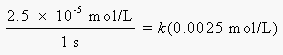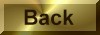Practice Problem 2

Calculate the rate constant for the reaction between phenolphthalein and the OH- ion if the instantaneous rate of reaction is 2.5 x 10-5 mole per liter per second when the concentration of phenolphthalein is 0.0025 M.

Solution

Rate = k(phenolphthalein)

We then substitute the known rate of reaction and the known concentration of phenolphthalein into this equation:Solving for the rate constant gives the following result:

k = 0.010 s-1